Introduction to Hardware Description Languages - 2

# Introduction to Hardware Description Languages - 2 - Embedded Systems (Web) - Computer Science Engineering (CSE)

1.3 Gate Level Modeling
In this level of abstraction the system modeling is done at the gate level ,i.e., the properties of the gates etc. to be used by the behavioral description of the system are defined. These definitions are known as primitives. Verilog has built in primitives for gates, transmission gates, switches, buffers etc.. These primitives are instantiated like modules except that they are predefined in verilog and do not need a module definition. Two basic types of gates are and/or gates & buf /not gates.

1.3.1 Gate Primitives

And/Or Gates: These have one scalar output and multiple scalar inputs. The output of the gate is evaluated as soon as the input changes .
wire OUT, IN1, IN2; // basic gate instantiations and a1(OUT, IN1, IN2); nand na1(OUT, IN1, IN2); or or1(OUT, IN1, IN2);

nor nor1(OUT, IN1, IN2); xor x1(OUT, IN1, IN2); xnor nx1(OUT, IN1, IN2); // more than two inputs; 3 input nand gate nand na1_3inp(OUT, IN1, IN2, IN3); // gate instantiation without instance name and (OUT, IN1, IN2); // legal gate instantiation

Buf/Not Gates: These gates however have one scalar input and multiple scalar outputs \// basic gate instantiations for bufif

bufif1 b1(out, in, ctrl);
bufif0 b0(out, in, ctrl);
// basic gate instantiations for notif
notif1 n1(out, in, ctrl);
notif0 n0(out, in, ctrl);

Array of instantiations

wire [7:0] OUT, IN1, IN2;
// basic gate instantiations
nand n_gate[7:0](OUT, IN1, IN2);

Gate-level multiplexer

A multiplexer serves a very efficient basic logic design element
// module 4:1 multiplexer
module mux4_to_1(out, i1, i2 , i3, s1, s0);
// port declarations output out; input i1, i2, i3; input s1, s0;
// internal wire declarations wire s1n, s0n; wire y0, y1, y2, y3 ;
//gate instantiations
// create s1n and s0n signals not (s1n, s1); not (s0n, s0);
// 3-input and gates instantiated
and (y0, i0, s1n, s0n);
and (y1, i1, s1n, s0); and (y2, i2, s1, s0n);
and (y3, i3, s1, s0);
// 4- input gate instantiated or (out, y0, y1, y2, y3);
endmodule

1.3.2 Gate and Switch delays
In real circuits, logic gates haves delays associated with them. Verilog provides the mechanism to associate delays with gates.
• Rise, Fall and Turn-off delays.
• Minimal, Typical, and Maximum delays

Rise Delay
The rise delay is associated with a gate output transition to 1 from another value (0,x,z)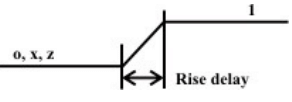Fall Delay

The fall delay is associated with a gate output transition to 0 from another value (1,x,z).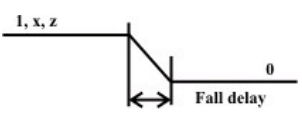Turn-off Delay

The Turn-off delay is associated with a gate output transition to z from another value (0,1,x).
Min Value
The min value is the minimum delay value that the gate is expected to have.
Typ Value
The typ value is the typical delay value that the gate is expected to have.
Max Value
The max value is the maximum delay value that the gate is expected to have.

1.4 Verilog Behavioral Modeling

1.4.1 Procedural Blocks

Verilog behavioral code is inside procedures blocks, but there is an exception, some behavioral code also exist outside procedures blocks. We can see this in detail as we make progress.

There are two types of procedural blocks in Verilog
• initial : initial blocks execute only once at time zero (start execution at time zero).
• always : always blocks loop to execute over and over again, in other words as the name means, it executes always.

Example – initial module initial_example();
reg clk,reset,enable,data; initial begin clk = 0;
reset = 0;
enable = 0;
data = 0; end
endmodule

In the above example, the initial block execution and always block execution starts at time 0. Always blocks wait for the the event, here positive edge of clock, where as initial block without waiting just executes all the statements within begin and end statement.

Example – always module always_example();
reg clk,reset,enable,q_in,data;
always @ (posedge clk) if (reset) begin data <= 0;
end else if (enable) begin data <= q_in;
end
endmodule

In always block, when the trigger event occurs, the code inside begin and end is executed and then once again the always block waits for next posedge of clock. This process of waiting and executing on event is repeated till simulation stops.

1.4.2 Procedural Assignment Statements

• Procedural assignment statements assign values to reg , integer , real , or time variables and can not assign values to nets ( wire data types)
• You can assign to the register (reg data type) the value of a net (wire), constant, another register, or a specific value.

1.4.3 Procedural Assignment Groups
If a procedure block contains more then one statement, those statements must be enclosed within Sequential begin - end block
• Parallel fork - join block
Example - "begin-end"
module
initial_begin_end();
reg clk,reset,enable,data;
initial begin
#1 clk = 0;
#10 reset = 0;
#5 enable = 0;
#3 data = 0;
end
endmodule

Begin : clk gets 0 after 1 time unit, reset gets 0 after 6 time units, enable after 11 time units, data after 13 units.
All the statements are executed sequentially.

Example - "fork-join"
module initial_fork_join();
reg clk,reset,enable,data;
initial fork #1 clk = 0; #10 reset = 0;
#5 enable = 0;
#3 data = 0;
join
endmodule

1.4.4 Sequential Statement Groups

The begin - end keywords:
• Group several statements together
• Cause the statements to be evaluated sequentially (one at a time)
• Any timing within the sequential groups is relative to the previous statement.
• Delays in the sequence accumulate (each delay is added to the previous delay)
•Block finishes after the last statement in the block.

1.4.5 Parallel Statement Groups

The fork - join keywords:
• Group several statements together.
• Cause the statements to be evaluated in parallel ( all at the same time).
• Timing within parallel group is absolute to the beginning of the group.
•  Block finishes after the last statement completes( Statement with high delay, it can be the first statement in the block)

Example – Parallel
module parallel();

reg a;
initial
fork
#10 a = 0;’
#11 a = 1; #12 a = 0;
#13 a = 1;
#14 a = \$finish;
join
endmodule

Example - Mixing "begin-end" and "fork - join"
module fork_join();
reg clk,reset,enable,data; initial begin \$display ( "Starting simulation" );
fork : FORK_VAL #1 clk = 0;
#5 reset = 0; #5 enable = 0;
#2 data = 0;
join \$display ( "Terminating simulation" );
#10 \$finish;
end
endmodule

1.4.6 Blocking and Nonblocking assignment
Blocking assignments are executed in the order they are coded, Hence they are sequential. Since they block the execution of the next statement, till the current statement is executed, they are called blocking assignments. Assignment are made with "=" symbol. Example a = b; Nonblocking assignments are executed in parallel. Since the execution of next statement is not blocked due to execution of current statement, they are called nonblocking statement. Assignment are made with "<=" symbol. Example a <= b;

Example - blocking and nonblocking
module blocking_nonblocking();
reg a, b, c, d ;
// Blocking Assignment initial begin #10 a = 0;
’ #11 a = 1;
#12 a = 0;
#13 a = 1;
end initial begin #10 b <= 0;
#11 b <=1; #12 b <=0;
#13 b <=1;
end initial begin c = #10 0;
c = #11 1;

c = #12 0;
c = #13 1;
end
initial begin
d <= #10 0;
d <= #11 1;
d <= #12 0;
d <= #13 1;
end
initial begin
\$monitor( " TIME = %t A = %b B = %b C = %b D = %b" ,\$time, a, b, c, d );
#50 \$finish(1);
end
endmodule

1.4.7 The Conditional Statement if-else
The if - else statement controls the execution of other statements. In programming language like c, if - else controls the flow of program. When more than one statement needs to be executed for an if conditions, then we need to use begin and end as seen in earlier examples.

Syntax: if if (condition) statements;
Syntax: if-else if (condition) statements;
else statements;

1.4.8 Syntax: nested if-else-if
if (condition) statements;
............... ................
else if (condition) statements;
else statements;

Example- simple
if module simple_if();
reg latch; wire enable,din;
always @ (enable or din) if (enable) begin latch <= din;
end
endmodule
Example- if-else
module if_else();

reg dff; wire clk,din,reset;
always @ (posedge clk) if (reset) begin dff <= 0;
end else begin dff <= din;
end
endmodule

Example- nested-if-else-if
module nested_if();
reg [3:0] counter; wire clk,reset,enable, up_en, down_en;
always @ (posedge clk)
// If reset is asserted if (reset == 1'b0) begin counter <= 4'b0000;
// If counter is enable and up count is mode end else if (enable == 1'b1 && up_en == 1'b1) begin counter <= counter + 1'b1;
// If counter is enable and down count is mode end else if (enable == 1'b1 && down_en == 1'b1) begin counter <= counter - 1'b0;
// If counting is disabled end else begin

counter <= counter; // Redundant code
end
endmodule

Parallel if-else

In the above example, the (enable == 1'b1 && up_en == 1'b1) is given highest pritority and condition (enable == 1'b1 && down_en == 1'b1) is given lowest priority. We normally don't include reset checking in priority as this does not fall in the combo logic input to the flip-flop as shown in figure below.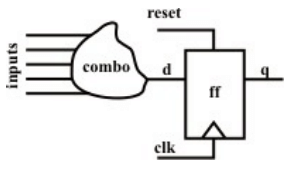So when we need priority logic, we use nested if-else statements. On the other end if we don't want to implement priority logic, knowing that only one input is active at a time i.e. all inputs are mutually exclusive, then we can write the code as shown below. It is a known fact that priority implementation takes more logic to implement then parallel implementation. So if you know the inputs are mutually exclusive, then you can code the logic in parallel if.

module parallel_if();
reg [3:0] counter; wire clk,reset,enable, up_en, down_en; always @ (posedge clk)
// If reset is asserted if (reset == 1'b0) begin counter <= 4'b0000; end else begin
// If counter is enable and up count is mode if (enable == 1'b1 && up_en == 1'b1) begin counter <= counter + 1'b1;
end
// If counter is enable and down count is mode if (enable == 1'b1 && down_en == 1'b1) begin counter <= counter - 1'b0;
end
end
endmodule

1.4.9 The Case Statement
The case statement compares an expression with a series of cases and executes the statement or statement group associated with the first matching case
• case statement supports single or multiple statements.
• Group multiple statements using begin and end keywords.
Syntax of a case statement look as shown below.
case ()
< case1 > : < statement >
< case2 > : < statement >
default :< statement >
endcase

1.4.10 Looping Statements
Looping statements appear inside procedural blocks only. Verilog has four looping statements like any other programming language.
• forever
• repeat
• while
• for

The forever statement The forever loop executes continually, the loop never ends. Normally we use forever statement in initial blocks.
syntax : forever < statement >

Once should be very careful in using a forever statement, if no timing construct is present in the forever statement, simulation could hang.
The repeat statement The repeat loop executes statement fixed < number > of times.
syntax : repeat (< number >) (< statement >)

The while loop statement The while loop executes as long as an evaluates as true. This is same as in any other programming language.
syntax: while (expression)
The for loop statement The for loop is same as the for loop used in any other programming language.
• Executes an < initial assignment > once at the start of the loop
• Executes the loop as long as an < expression > evaluates as true.
• Executes a at the end of each pass through the loop
syntax : for (< initial assignment >; < expression >, < step assignment >) < statement >
Note : verilog does not have ++ operator as in the case of C language.

1.5 Switch level modeling
1.5.1 Verilog provides the ability to design at MOS-transistor level, however with increase in complexity of the circuits design at this level is growing tough. Verilog however only provides digital design capability and drive strengths associated to them. Analog capability is not into picture still. As a matter of fact transistors are only used as switches.
MOS switches
//MOS switch keywords nmos pmos
Whereas the keyword nmos is used to model a NMOS transistor, pmos is used for PMOS transistors.

Instantiation of NMOS and PMOS switches nmos n1(out, data, control);
// instantiate a NMOS switch pmos p1(out, data, control);
// instantiate a PMOS switch

CMOS switches
Instantiation of a CMOS switch.
cmos c1(out, data, ncontrol, pcontrol );
// instantiate a cmos switch
The ‘ncontrol’ and ‘pcontrol’ signals are normally complements of each other

Bidirectional switches
These switches allow signal flow in both directions and are defined by keywords tran,tranif0 , and tranif1

Instantiation
tran t1(inout1, inout2); // instance name t1 is optional
tranif0(inout1, inout2, control); // instance name is not specified
tranif1(inout1, inout2, control); // instance name t1 is not specified

1.5.2 Delay specification of switches
pmos, nmos, rpmos, rnmos
• Zero(no delay) pmos p1(out,data, control);
• One (same delay in all) pmos#(1) p1(out,data, control);
• Two(rise, fall) nmos#(1,2) n1(out,data, control);
• Three(rise, fall, turnoff)mos#(1,3,2) n1(out,data,control);

1.5.3 An Instance: Verilog code for a NOR- gate
// define a nor gate, my_nor
module my_nor(out, a, b);
output out;
input a, b;
//internal wires
wire c;
// set up pwr n ground lines
supply1 pwr;// power is connected to vdd
supply0 gnd; // connected to Vss
// instantiate pmos switches pmos (c, pwr, b);
pmos (out, c, a);
//instantiate nmos switches
nmos (out, gnd, a);
Stimulus to test the NOR-gate
// stimulus to test the gate
module stimulus;
reg A, B;
wire OUT;
//instantiate the my_nor module my_nor n1(OUT, A, B);
//Apply stimulus
initial
begin
//test all possible combinations A=1’b0;
B=1’b0;
#5 A=1’b0;B=1’b1;
#5 A=1’b1; B=1’b0;
#5 A=1’b1;B=1’b1;
end //check results
initial
\$ monitor(\$time, “OUT = %b, B=%b, OUT, A, B);
endmodule

1.6 Some Exercises
1.6.1 Gate level modelling

i) A 2 inp xor gate can be build from my_and, my_or and my_not gates. Construct an xor module in verilog that realises the logic function z= xy'+x'y. Inputs are x, y and z is the output. Write a stimulus module that exercises all the four combinations of x and y
ii) The logic diagram for an RS latch with delay is being shown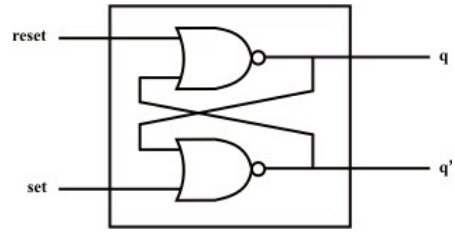Write the verilog description for the RS latch, including delays of 1 unit when instantiating the nor gates. Write the stimulus module for the RS latch using the following table and verify the output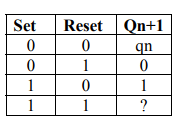iii) Design a 2-input multiplexer using bufif0 and bufif1 gates as shown below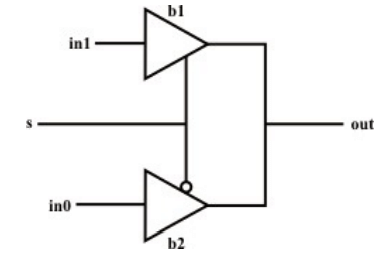The delay specification for gates b1 and b2 are as follows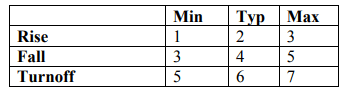1.6.2. Behavioral modelling i) Using a while loop design a clk generator whose initial value is 0. time period of the clk is 10.
ii) Using a forever statement, design a clk with time period=10 and duty cycle =40%. Initial value of clk is 0
iii) Using the repeat loop, delay the statement a=a+1 by 20 positive edges of clk.
iv) Design a negative edge triggered D-FF with synchronous clear, active high (D-FF clears only at negative edge of clk when clear is high). Use behavioral statements only. (Hint: output q of DFF must be declared as reg.) Design a clock with a period of 10units and test the D-FF v) Design a 4 to 1 multiplexer using if and else statements vi) Design an 8-bit counter by using a forever loop, named block, and disabling of named block. The counter starts counting at count =5 and finishes at count =67. The count is incremented at positive edge of clock. The clock has a time period of 10. The counter starts through the loop only once and then is disabled (hint: use the disable statement)

The document Introduction to Hardware Description Languages - 2 | Embedded Systems (Web) - Computer Science Engineering (CSE) is a part of the Computer Science Engineering (CSE) Course Embedded Systems (Web).
All you need of Computer Science Engineering (CSE) at this link: Computer Science Engineering (CSE)

## Embedded Systems (Web)

47 videos|69 docs|65 tests

## Embedded Systems (Web)

47 videos|69 docs|65 tests
Signup to see your scores go up within 7 days! Learn & Practice with 1000+ FREE Notes, Videos & Tests.
10M+ students study on EduRev
Track your progress, build streaks, highlight & save important lessons and more!(Scan QR code)
Related Searches

,

,

,

,

,

,

,

,

,

,

,

,

,

,

,

,

,

,

,

,

,

;